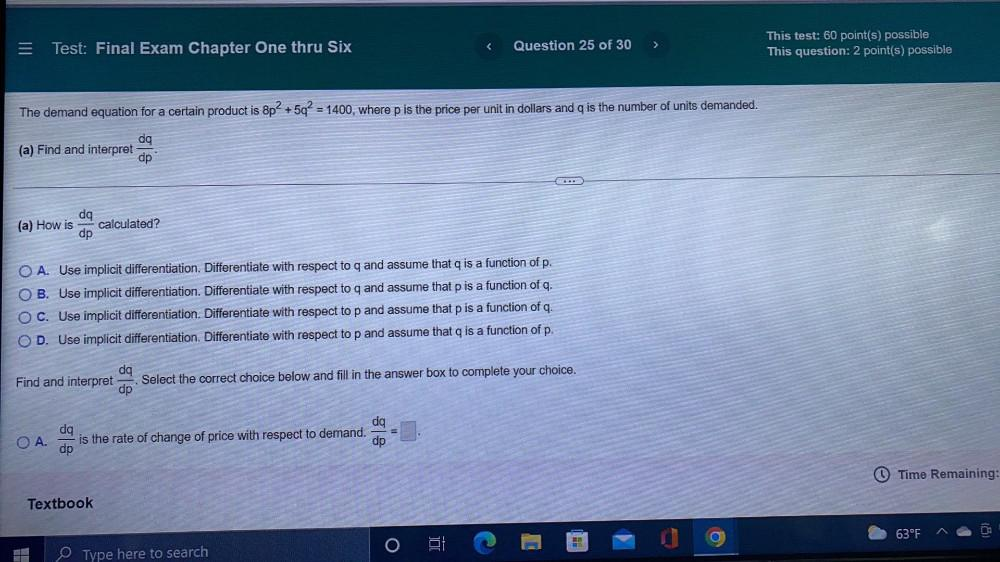Question:

# The demand equation for a certain product is 8p² + 5q² = 1400, where p is the price per unit in dollars and is the number of units demanded. (a) Find and interpret dq/dp. (a) How is dq/dp calculated?The demand equation for a certain product is 8p² + 5q² = 1400, where p is the price per unit in dollars and is the number of units demanded. (a) Find and interpret dq/dp. (a) How is dq/dp calculated? OA. Use implicit differentiation. Differentiate with respect to q and assume that q is a function of p. OB. Use implicit differentiation. Differentiate with respect to q and assume that p is a function of q. O C. Use implicit differentiation. Differentiate with respect to p and assume that p is a function of q. OD. Use implicit differentiation. Differentiate with respect to p and assume that q is a function of p. Find and interpret dq/dp. Select the correct choice below and fill in the answer box to complete your choice. ○ A. dq/dp is the rate of change of price with respect to demand dq/dp = ▢.